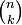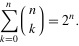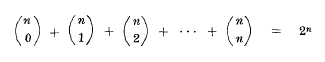# binomial coefficients

Warning: This blogpost has been posted over two years ago. That is a long time in development-world! The story here may not be relevant, complete or secure. Code might not be complete or obsoleted, and even my current vision might have (completely) changed on the subject. So please do read further, but use it with caution.Posted on 10 Apr 2011
Tagged with: [ coefficients ]  [ math ]  [ pascals triangle

Question: how can a simple question asked by a colleague turn into a blog post? Answer: when he asked: how many different queries can I build when I have 270 fields? The answer to solve this problem: binomial coefficients.

Now I do enjoy math. I’m definitely no guru in math but I (hopefully) know a thing or two. Just like with programming and everything computer related: if you know something vaguely, at least you know enough to look up the actual application for it. Our first instinct on the answer to my colleague was 270! (factorial of 270, which is 1x2x3x4x…x267x270, which is a VERY large number) but I knew something was not right. Turns out: it wasn’t right. The problem was mixing up combinations and permutations, which I knew there was a big difference, but I didn’t knew the math involved anymore..

## Combinations and permutations

Combinations and permutations are two different ways which are mixed up by a lot of people. Suppose we have a set of elements, say, the alphabet. Thus alphabet consists of 26 different elements {A, B,…Z }. Now suppose we want to know how many 4-letter “words” we can create (they don’t have to be existing words, everything with 4 letters is ok). But before we can answer that, we must know 2 things:

1. does the order matter? Is ABCD the same as DCBA or ACDB?
2. can we have repetitive elements? Is AABC allowed? or even ZZZZ?

With the first question we make a distinction between a combination and permutation. A combination does not care about the actual order, but a permutation does. So the combination ABCD is the same as the combination DCBA. However, the permutation ABCD and DCBA are different. As you might guess, there always be more possible permutations than combinations when dealing with sets.

Now the second question tells us if the combination (or permutation) is repetitive or not. When we can have items like AABC, many more combinations or permutations are possible. Some sets can have repetitive elements, like when creating words from an alphabet, but some arent. For instance, pulling 10 cards from a deck of cards. There will be only 1 ace of spades inside the deck, so there can be no repetitive combination or permutation.

### Permutations

Calculating the number of permutations is easy and maybe the most used, which is why we all yelled out the answer to my collegue without thinking about the actual problem. We can actually use the factorial of n, where n is the number of elements or in mathemical terms: n! These are non-repetative permutations so things like AAAA or AAAB are not allowed.Since there are 270 different elements to choose from, 270! would have been the answer (which is very very large, even google doesn’t let us display it. The largest number google will display is 170! (which is 7.257 x 10306, so there)…

Now since we were dealing with query fields (either present, or not), this answer was actually wrong. Having a query like “q=element1&element2&element3” is the same as “q=element3&element2&element1”. So we need to deal with combinations and not permutations.

Also, items are non-repetitive. We cannot have a “q=element1&element1&element2”. If it was, our formula should at least have been nr. Where r stands for the number of items we need to select, which in our case is the same as n since we need to select 270 elements from the set of 270 elements.

## Combinations

Combinations are a bit harder to calculate, since order does not matter. When we get all non-repetative permutations from a set of `{ 1, 2, 3 }` we get `3!` which is `1x2x3 = 6  {123, 132, 213, 231, 312, 321 }`. But the order does not matter in combinations, so we should only have 1 possible combination here, namely `{1,2,3}`, since we want to pick 3 elements out of the set of 3 elements. But when we need 2 elements from the set, how many combinations are possible? Answer by brute force: 3, namely `{1,2}`, `{1,3}` and `{2,3}`. Easy enough to find out with a pen and piece of paper.

This formula is called the binomial coefficient and the formula is:Theis just an easier way of writing the formula. Turns out math-people are just as lazy as programmers :) There are other variations but this is the most common used. Now this formula is nothing more on saying that if we have “n” items how many combinations of “k” items can we make?

So in our example above we had n = 3, and k = 2.  When filling it in our function we get ```(3! / (2! . (3-2)!)) = 6 / (2 . 1) = 3```

It works with selecting 3 elements as wel: ` 3! / (3! . 0!) = 6 / (6 . 1) = 1`, which is the only combination possible as we have seen before.

The number we get is actually the number of distinct “k-subsets” it has. So with n = 3 and k = 2 we have 3 possible k-subsets ({1,2}, {1,3} and {2,3}).

Now, it’s easy enough to remember that when we have a set of n elements, the number of combinations possible when picking n elements is actually 1. But we are not picking only n elements. Turns out, the query from my colleague can have an unspecified number elements. It can have only 1 element, maybe 2, maybe 3 up to a maximum of 270 elements and it’s even possible to have no elements as well, but none of them are repetitive.

So we have to use our formula for every k ranging from 0 to 270 (n always stays the same). So more or less like we have a for() loop in php, we do have the same thing on mathematical formula’s:The big “∑” thing stands for summation, ranging from k=0 (bottom of the ∑), to n (top of the ∑). The (n k) thing inside the brackets is the same formula as above, written in short notation.

Now, when we count all the possible results, we actually find that it’s the same as using 2n. This is because we are doing this:which in the end can be rewritten to 2n.

So when we have 3 elements {ABC}, we can have 23 (8) different k-subsets: {-, 1, 2, 3, 12, 13, 23, 123}. The - means “no elements”, which off course is a valid subset as well, since k can be 0.

## Conclusion

There is a big difference between permutations and combinations. They are not the same although most people probably will call them both combinations. It does has some practical applicances as well as I’ve shown you, but how about storage? Maybe it’s ok to store the combinations, instead of permutations? Is the order in which people do a query important? If not, it can save you time and space to log only the combinations instead of the permutations.

So all this stuff is needed to getting to our answer: 2n = 2270 = 1.89713759 x 1081. Which is less than 270! of course, but still a big number. So in the end my collegue got his answer, and I got a new blogpost :)